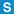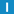# class FPEnvironment

Library: Foundation
Package: Core

## Description

Instances of this class can be used to save and later restore the current floating point environment (consisting of rounding mode and floating-point flags). The class also provides various static methods to query certain properties of a floating-point number.

## Inheritance

Direct Base Classes: FPEnvironmentImpl

All Base Classes: FPEnvironmentImpl

## Member Summary

Member Functions: clearFlags, copySign, getRoundingMode, isFlag, isInfinite, isNaN, keepCurrent, operator =, setRoundingMode

## Enumerations

### Flag

FP_DIVIDE_BY_ZERO = FP_DIVIDE_BY_ZERO_IMPL

FP_INEXACT = FP_INEXACT_IMPL

FP_OVERFLOW = FP_OVERFLOW_IMPL

FP_UNDERFLOW = FP_UNDERFLOW_IMPL

FP_INVALID = FP_INVALID_IMPL

### RoundingMode

FP_ROUND_DOWNWARD = FP_ROUND_DOWNWARD_IMPL

FP_ROUND_UPWARD = FP_ROUND_UPWARD_IMPL

FP_ROUND_TONEAREST = FP_ROUND_TONEAREST_IMPL

FP_ROUND_TOWARDZERO = FP_ROUND_TOWARDZERO_IMPL

## Constructors

### FPEnvironment

Standard constructor. Remembers the current environment.

### FPEnvironment

FPEnvironment(
RoundingMode mode
);

Remembers the current environment and sets the given rounding mode.

### FPEnvironment

FPEnvironment(
const FPEnvironment & env
);

Copy constructor.

## Destructor

### ~FPEnvironment

~FPEnvironment();

Restores the previous environment (unless keepCurrent() has been called previously)

## Member Functions

### clearFlagsstatic void clearFlags();

Resets all flags.

### copySignstatic float copySign(
float target,
float source
);

### copySignstatic double copySign(
double target,
double source
);

### copySignstatic long double copySign(
long double target,
long double source
);

Copies the sign from source to target.

### getRoundingModestatic RoundingMode getRoundingMode();

Returns the current rounding mode.

### isFlagstatic bool isFlag(
Flag flag
);

Returns true if and only if the given flag is set.

### isInfinitestatic bool isInfinite(
float value
);

### isInfinitestatic bool isInfinite(
double value
);

### isInfinitestatic bool isInfinite(
long double value
);

Returns true if and only if the given number is infinite.

### isNaNstatic bool isNaN(
float value
);

### isNaNstatic bool isNaN(
double value
);

### isNaNstatic bool isNaN(
long double value
);

Returns true if and only if the given number is NaN.

### keepCurrent

void keepCurrent();

Keep the current environment even after destroying the FPEnvironment object.

### operator =

FPEnvironment & operator = (
const FPEnvironment & env
);

Assignment operator

### setRoundingModestatic void setRoundingMode(
RoundingMode mode
);

Sets the rounding mode.